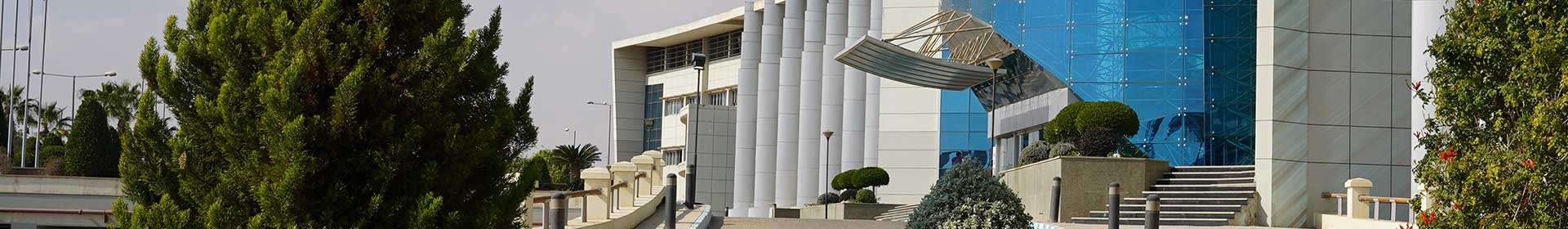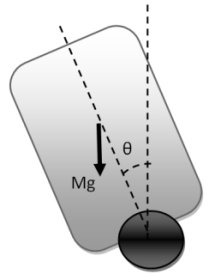Conference Paper

# Two-wheeled Self balancing robot Modeling and Control using Artificial Neural Networks (ANN)

By
Omar H.M.
Elalawy A.M.
Ammar H.H.

This paper is focusing on the problem of the Self balancing robot which has many potentials due to its power consumption and maneuverability advantages. Modelling and controlling of the two-wheeled self-balancing robot is presented. For modelling part, two models were used in compare with a real proposed robot. At first, mathematical model was driven and the state space was achieved to model the plant of the system. Second, Nonlinear Autoregressive Exogenous (NARX) Neural Network model is introduced using recorded data architecture-based as it is used in time-series modeling for many reported nonlinear systems. The results proved that NARX provides a better model over the mathematical one. For controlling part, we are presenting a comparison between two different controlling architectures: PID and Model Reference Adaptive Control (MRAC). The two proposed controllers were found suitable solutions in this control problem. However, MRAC gives the best results in following random inputs and is favored in disturbance rejection, then comes the PID controller. © 2019 IEEE.